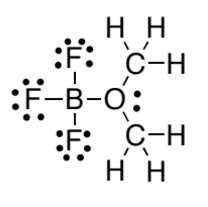# Problem: In the following structure, what are the formal charges on boron? A. B =  1+ B. B = 1– C. B = 0

###### FREE Expert Solution
96% (428 ratings)
###### Problem Details

In the following structure, what are the formal charges on boron?

A. B =  1+

B. B = 1

C. B = 0What scientific concept do you need to know in order to solve this problem?

Our tutors have indicated that to solve this problem you will need to apply the Formal Charges concept. You can view video lessons to learn Formal Charges. Or if you need more Formal Charges practice, you can also practice Formal Charges practice problems.

What is the difficulty of this problem?

Our tutors rated the difficulty ofIn the following structure, what are the formal charges on b...as low difficulty.

How long does this problem take to solve?

Our expert Organic tutor, Chris took 1 minute and 32 seconds to solve this problem. You can follow their steps in the video explanation above.

What professor is this problem relevant for?

Based on our data, we think this problem is relevant for Professor Modarelli's class at UAKRON.